# Resources tagged with: Place value

Filter by: Content type:
Age range:
Challenge level:

### There are 75 results

Broad Topics > Numbers and the Number System > Place value### Basically

##### Age 11 to 14 Challenge Level:

The number 3723(in base 10) is written as 123 in another base. What is that base?### Double Digit

##### Age 11 to 14 Challenge Level: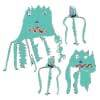### Back to the Planet of Vuvv

##### Age 11 to 14 Challenge Level:

There are two forms of counting on Vuvv - Zios count in base 3 and Zepts count in base 7. One day four of these creatures, two Zios and two Zepts, sat on the summit of a hill to count the legs of. . . .##### Age 7 to 14 Challenge Level:

Watch our videos of multiplication methods that you may not have met before. Can you make sense of them?### Lesser Digits

##### Age 11 to 14 Challenge Level:

How many positive integers less than or equal to 4000 can be written down without using the digits 7, 8 or 9?### Even Up

##### Age 11 to 14 Challenge Level:

Consider all of the five digit numbers which we can form using only the digits 2, 4, 6 and 8. If these numbers are arranged in ascending order, what is the 512th number?### Eleven

##### Age 11 to 14 Challenge Level:

Replace each letter with a digit to make this addition correct.### Alien Counting

##### Age 7 to 11 Challenge Level:

Investigate the different ways these aliens count in this challenge. You could start by thinking about how each of them would write our number 7.##### Age 11 to 18

We are used to writing numbers in base ten, using 0, 1, 2, 3, 4, 5, 6, 7, 8, and 9. Eg. 75 means 7 tens and five units. This article explains how numbers can be written in any number base.### Arrange the Digits

##### Age 11 to 14 Challenge Level:

Can you arrange the digits 1,2,3,4,5,6,7,8,9 into three 3-digit numbers such that their total is close to 1500?### Football Sum

##### Age 11 to 14 Challenge Level:

Find the values of the nine letters in the sum: FOOT + BALL = GAME### Digit Sum

##### Age 11 to 14 Challenge Level:

What is the sum of all the digits in all the integers from one to one million?### More Mathematical Mysteries

##### Age 11 to 14 Challenge Level:

Write down a three-digit number Change the order of the digits to get a different number Find the difference between the two three digit numbers Follow the rest of the instructions then try. . . .### How Many Miles to Go?

##### Age 11 to 14 Challenge Level:

How many more miles must the car travel before the numbers on the milometer and the trip meter contain the same digits in the same order?### Cayley

##### Age 11 to 14 Challenge Level:

The letters in the following addition sum represent the digits 1 ... 9. If A=3 and D=2, what number is represented by "CAYLEY"?### Quick Times

##### Age 11 to 14 Challenge Level:

32 x 38 = 30 x 40 + 2 x 8; 34 x 36 = 30 x 40 + 4 x 6; 56 x 54 = 50 x 60 + 6 x 4; 73 x 77 = 70 x 80 + 3 x 7 Verify and generalise if possible.### Which Is Quicker?

##### Age 7 to 11 Challenge Level:

Which is quicker, counting up to 30 in ones or counting up to 300 in tens? Why?### What Do You Need?

##### Age 7 to 11 Challenge Level:

Four of these clues are needed to find the chosen number on this grid and four are true but do nothing to help in finding the number. Can you sort out the clues and find the number?### Skeleton

##### Age 11 to 14 Challenge Level:

Amazing as it may seem the three fives remaining in the following `skeleton' are sufficient to reconstruct the entire long division sum.##### Age 11 to 14 Challenge Level:

Powers of numbers behave in surprising ways. Take a look at some of these and try to explain why they are true.### Six Times Five

##### Age 11 to 14 Challenge Level:

How many six digit numbers are there which DO NOT contain a 5?### Subtraction Surprise

##### Age 7 to 14 Challenge Level:

Try out some calculations. Are you surprised by the results?### The Number Jumbler

##### Age 7 to 14 Challenge Level:

The Number Jumbler can always work out your chosen symbol. Can you work out how?### Always a Multiple?

##### Age 11 to 14 Challenge Level:

Think of a two digit number, reverse the digits, and add the numbers together. Something special happens...### Becky's Number Plumber

##### Age 7 to 11 Challenge Level:

Becky created a number plumber which multiplies by 5 and subtracts 4. What do you notice about the numbers that it produces? Can you explain your findings?### A Story about Absolutely Nothing

##### Age 7 to 18

This article for the young and old talks about the origins of our number system and the important role zero has to play in it.### Mini-max

##### Age 11 to 14 Challenge Level:

Consider all two digit numbers (10, 11, . . . ,99). In writing down all these numbers, which digits occur least often, and which occur most often ? What about three digit numbers, four digit numbers. . . .### Seven Up

##### Age 11 to 14 Challenge Level:

The number 27 is special because it is three times the sum of its digits 27 = 3 (2 + 7). Find some two digit numbers that are SEVEN times the sum of their digits (seven-up numbers)?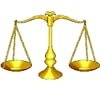### Balance Power

##### Age 11 to 18 Challenge Level:

Using balancing scales what is the least number of weights needed to weigh all integer masses from 1 to 1000? Placing some of the weights in the same pan as the object how many are needed?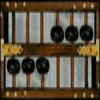##### Age 5 to 16

Nowadays the calculator is very familiar to many of us. What did people do to save time working out more difficult problems before the calculator existed?### Chocolate Maths

##### Age 11 to 14 Challenge Level:

Pick the number of times a week that you eat chocolate. This number must be more than one but less than ten. Multiply this number by 2. Add 5 (for Sunday). Multiply by 50... Can you explain why it. . . .### The Thousands Game

##### Age 7 to 11 Challenge Level:

Each child in Class 3 took four numbers out of the bag. Who had made the highest even number?### Being Resilient - Primary Number

##### Age 5 to 11 Challenge Level:

Number problems at primary level that may require resilience.### Big Powers

##### Age 11 to 16 Challenge Level:

Three people chose this as a favourite problem. It is the sort of problem that needs thinking time - but once the connection is made it gives access to many similar ideas.### Song Book

##### Age 7 to 11 Challenge Level:

A school song book contains 700 songs. The numbers of the songs are displayed by combining special small single-digit cards. What is the minimum number of small cards that is needed?##### Age 5 to 11 Challenge Level:

Who said that adding couldn't be fun?##### Age 11 to 14 Challenge Level:

Replace the letters with numbers to make the addition work out correctly. R E A D + T H I S = P A G E### Not a Polite Question

##### Age 11 to 14 Challenge Level:

When asked how old she was, the teacher replied: My age in years is not prime but odd and when reversed and added to my age you have a perfect square...### X Marks the Spot

##### Age 11 to 14 Challenge Level:

When the number x 1 x x x is multiplied by 417 this gives the answer 9 x x x 0 5 7. Find the missing digits, each of which is represented by an "x" .### Three Times Seven

##### Age 11 to 14 Challenge Level:

A three digit number abc is always divisible by 7 when 2a+3b+c is divisible by 7. Why?### Repeaters

##### Age 11 to 14 Challenge Level:

Choose any 3 digits and make a 6 digit number by repeating the 3 digits in the same order (e.g. 594594). Explain why whatever digits you choose the number will always be divisible by 7, 11 and 13.### Legs Eleven

##### Age 11 to 14 Challenge Level:

Take any four digit number. Move the first digit to the end and move the rest along. Now add your two numbers. Did you get a multiple of 11?### What an Odd Fact(or)

##### Age 11 to 14 Challenge Level:

Can you show that 1^99 + 2^99 + 3^99 + 4^99 + 5^99 is divisible by 5?### Cycle It

##### Age 11 to 14 Challenge Level:

Carry out cyclic permutations of nine digit numbers containing the digits from 1 to 9 (until you get back to the first number). Prove that whatever number you choose, they will add to the same total.### Oddly

##### Age 7 to 11 Challenge Level:

Find the sum of all three-digit numbers each of whose digits is odd.### Tis Unique

##### Age 11 to 14 Challenge Level:

This addition sum uses all ten digits 0, 1, 2...9 exactly once. Find the sum and show that the one you give is the only possibility.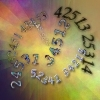### Permute It

##### Age 11 to 14 Challenge Level:

Take the numbers 1, 2, 3, 4 and 5 and imagine them written down in every possible order to give 5 digit numbers. Find the sum of the resulting numbers.##### Age 5 to 11 Challenge Level:

Try out this number trick. What happens with different starting numbers? What do you notice?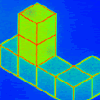### Pupils' Recording or Pupils Recording

##### Age 5 to 14

This article, written for teachers, looks at the different kinds of recordings encountered in Primary Mathematics lessons and the importance of not jumping to conclusions!### Napier's Bones

##### Age 7 to 11 Challenge Level:

The Scot, John Napier, invented these strips about 400 years ago to help calculate multiplication and division. Can you work out how to use Napier's bones to find the answer to these multiplications?